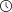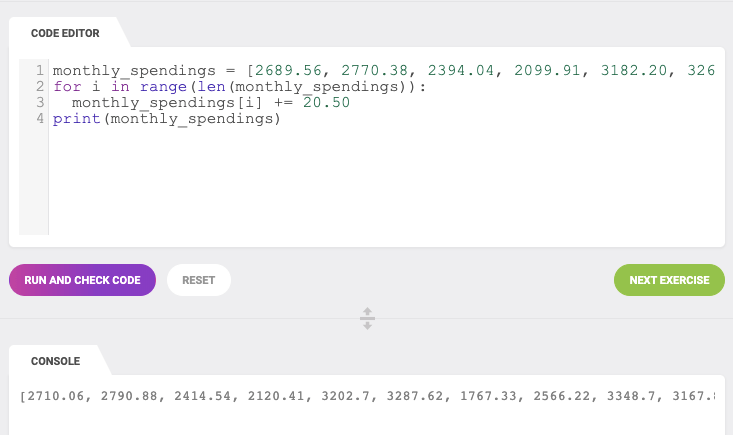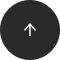- 5 minutes read

# Array vs. List in Python – What's the Difference?

Both lists and arrays are used to store data in Python. Moreover, both data structures allow indexing, slicing, and iterating. So what's the difference between an array and a list in Python? In this article, we'll explain in detail when to use a Python array vs. a list.

Python has lots of different data structures with different features and functions. Its built-in data structures include lists, tuples, sets, and dictionaries. However, this is not an exhaustive list of the data structures available in Python. Some additional data structures can be imported from different modules or packages.

An array data structure belongs to the "must-import" category. To use an array in Python, you'll need to import this data structure from the NumPy package or the array module.

And that's the first difference between lists and arrays. Before diving deeper into the differences between these two data structures, let's review the features and functions of lists and arrays.

### What Is a List in Python?

A list is a data structure that's built into Python and holds a collection of items. Lists have a number of important characteristics:

• List items are enclosed in square brackets, like this [item1, item2, item3].
• Lists are ordered – i.e. the items in the list appear in a specific order. This enables us to use an index to access to any item.
• Lists are mutable, which means you can add or remove items after a list's creation.
• List elements do not need to be unique. Item duplication is possible, as each element has its own distinct place and can be accessed separately through the index.
• Elements can be of different data types: you can combine strings, integers, and objects in the same list.

Lists are very easily created in Python:

```list = [3, 6, 9, 12]
print(list)
print(type(list))
```
```[3, 6, 9, 12]
<class 'list'>
```

Python lists are used just about everywhere, as they are a great tool for saving a sequence of items and iterating over it.

### What Is an Array in Python?

An array is also a data structure that stores a collection of items. Like lists, arrays are ordered, mutable, enclosed in square brackets, and able to store non-unique items.

But when it comes to the array's ability to store different data types, the answer is not as straightforward. It depends on the kind of array used.

To use arrays in Python, you need to import either an array module or a NumPy package.

```import array as arr
```
```import numpy as np
```

The Python array module requires all array elements to be of the same type. Moreover, to create an array, you'll need to specify a value type. In the code below, the "i" signifies that all elements in array_1 are integers:

```array_1 = arr.array("i", [3, 6, 9, 12])
print(array_1)
print(type(array_1))
```
```array('i', [3, 6, 9, 12])
<class 'array.array'>
```

On the other hand, NumPy arrays support different data types. To create a NumPy array, you only need to specify the items (enclosed in square brackets, of course):

```array_2 = np.array(["numbers", 3, 6, 9, 12])
print (array_2)
print(type(array_2))
```
```['numbers' '3' '6' '9' '12']
<class 'numpy.ndarray'>
```

As you can see, array_2 contains one item of the string type (i.e., "numbers") and four integers.

### So What's the Difference?

Now that we know their definitions and features, we can talk about the differences between lists and arrays in Python:

• Arrays need to be declared. Lists don't, since they are built into Python. In the examples above, you saw that lists are created by simply enclosing a sequence of elements into square brackets. Creating an array, on the other hand, requires a specific function from either the array module (i.e., array.array()) or NumPy package (i.e., numpy.array()). Because of this, lists are used more often than arrays.
• Arrays can store data very compactly and are more efficient for storing large amounts of data.
• Arrays are great for numerical operations; lists cannot directly handle math operations. For example, you can divide each element of an array by the same number with just one line of code. If you try the same with a list, you'll get an error.
```array = np.array([3, 6, 9, 12])
division = array/3
print(division)
print (type(division))
```
```[1. 2. 3. 4.]
<class 'numpy.ndarray'>
```
```list = [3, 6, 9, 12]
division = list/3
```
```---------------------------------------------------------------------------
TypeError                                 Traceback (most recent call last)
in ()
1 list = [3, 6, 9, 12]
----> 2 division = list/3

TypeError: unsupported operand type(s) for /: 'list' and 'int'
```

Of course, it's possible to do a mathematical operation with a list, but it's much less efficient:From the Python Data Structures in Practice course

So, when should you use a list and when should you use an array?

• If you need to store a relatively short sequence of items and you don't plan to do any mathematical operations with it, a list is the preferred choice. This data structure will allow you to store an ordered, mutable, and indexed sequence of items without importing any additional modules or packages.
• If you have a very long sequence of items, consider using an array. This structure offers more efficient data storage.
• If you plan to do any numerical operations with your combination of items, use an array. Data analytics and data science rely heavily on (mostly NumPy) arrays.

### Time to Practice Python Arrays and Lists!

Great! Now you know the difference between an array and a list in Python. You also know which to choose for a sequence of items. Now it's time to practice!

If you want to advance your understanding of data structures and practice 100+ interactive exercises, check out the LearnPython.com course Python Data Structures in Practice. It will help you feel like a pro when dealing with lists, nested lists, tuples, sets, and dictionaries.